The trap occupation probability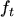can be calculated by examining the steady state in which the following relation holds2.12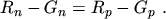(2.211)

For the non degenerated case, that is, for the FERMI level several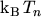below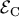,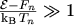, MAXWELL-BOLTZMANN statistics can be assumed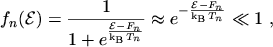(2.212)

which further allows to assume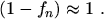(2.213)

Using the approximations (2.212) and (2.213) and the analog approximations for holes, equations (2.209) and (2.210) can be written as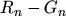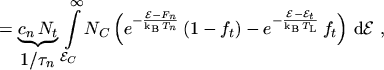(2.214)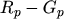(2.215)

where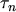and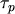are the lifetimes for electrons and holes, respectively. The characteristic parameters describing the interaction of carriers and trap centers are the capture cross sections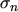and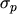. If they are known the rate constants (and thus also the lifetimes) can be expressed as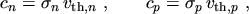(2.216)

where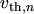and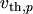are the thermal velocities of electrons and holes, respectively.

Using the following expressions for the electron and hole concentrations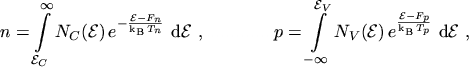(2.217)

and the handy abbreviations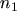and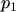for the electron and hole concentrations when the FERMI level is equal to the trap level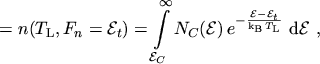(2.218)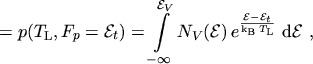(2.219)

eqns. (2.214) and (2.215) can be written as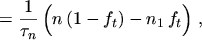(2.220)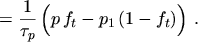(2.221)

Making use of the steady state condition eqn. (2.211) the following expression foris found(2.222)

Inserting eqn. (2.222) either in eqn. (2.214) or eqn. (2.215) yields the well known SHOCKLEY-READ-HALL net recombination rate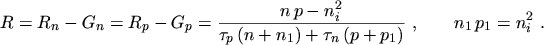(2.223)

M. Gritsch: Numerical Modeling of Silicon-on-Insulator MOSFETs PDF